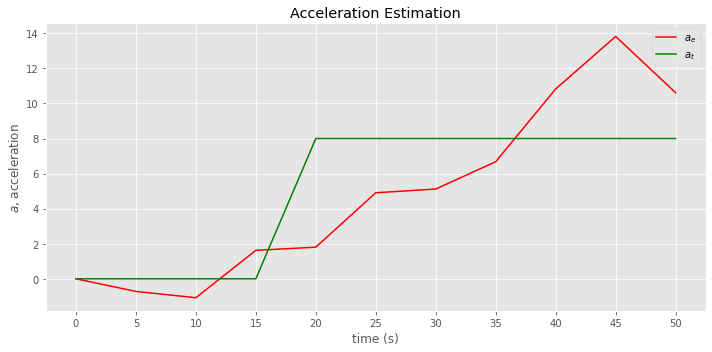# 13. Kalman Filter, I

Let’s study the Kalman Filter based on KalmanFilter.NET [Bec18].

## 13.1. Weighting Gold

This example is from the $$\alpha$$ - $$\beta$$ - $$\gamma$$ tutorial. In this example, we are weighting a gold bar, whose true weight is 1010 g. To start off, our final estimate $$\hat{x}_{N,N}$$ of the gold bar should be the average of all our measurements $$z_n$$.

$$\hat{x}_{N,N} = \frac{1}{N} \sum z_n$$

where

• $$N$$ is the number of measurements taken and can also be taken to mean time points

• $$z_n$$ is the measurement taken at time $$n$$

• $$\hat{x}_{N,N}$$ is the estimation of the weight based on all the measurements

This equation is fine if we want to take many measurements and then compute the final estimate in one swoop at the end. However, the Kalman Filter is meant to estimate the gold bar weight in real time. The State Update Equation provides the formula for estimating the gold bar weight as we take measurements (as opposed to one time only at the very end). The State Update Equation is written as follows.

$$\hat{x}_{n,n} = \hat{x}_{n,n-1} + \alpha_n (z_n - \hat{x}_{n,n-1})$$

• $$x$$ is the true value of the weight

• $$z_n$$ is the measurement at time $$n$$

• $$\hat{x}_{n,n}$$ is the estimate of $$x$$ at time $$n$$

• $$\hat{x}_{n,n-1}$$ is the previous estimate of $$x$$ at time $$n-1$$

• $$\hat{x}_{n+1,n}$$ is the next estimate of $$x$$ at time $$n+1$$

• $$\alpha_n$$ is called the Kalman Gain

• $$(z_n - \hat{x}_{n,n-1})$$ is called the innovation

$$\alpha_n$$ decreases as the number of iteration (measurements over time) grows, thereby weighting down the measurements $$z_n$$. Since the dynamic state of the system is constant (the gold weight does not change), $$\hat{x}_{n+1,n} = \hat{x}_{n,n}$$.

Below, is how we use the State Update Equation to estimate the gold bar weight over 10 measurements (in real-time).

:

import pandas as pd

measurements = [1030, 989, 1017, 1009, 1013, 979, 1008, 1042, 1012, 1011]
estimates = []
iters = []
x_ij = 1000

for i, z_i in enumerate(measurements):
n = i + 1
alpha_i = 1 / n
x_ii = x_ij + alpha_i * (z_i - x_ij)
x_ij = x_ii

iters.append(n)
estimates.append(x_ii)

measurements = pd.Series(measurements, index=iters)
estimates = pd.Series(estimates, index=iters)


Let’s plot the measurements, estimations and true gold bar weight.

:

import matplotlib.pyplot as plt
import numpy as np

np.random.seed(37)
plt.style.use('ggplot')

fig, ax = plt.subplots(figsize=(10, 3))

_ = measurements.plot(kind='line', ax=ax, color='blue', label='measurement')
_ = estimates.plot(kind='line', ax=ax, color='red', label='estimate')
_ = ax.axhline(1010, color='green', alpha=0.5, label='true')
_ = ax.set_xticks(range(1, len(measurements) + 1))
_ = ax.set_xticklabels(measurements.index)
_ = ax.legend()
_ = ax.set_xlabel('iterations')
_ = ax.set_ylabel('weight (g)')
_ = ax.set_title('Kalman Filter, State Update Equation, Weighting Gold')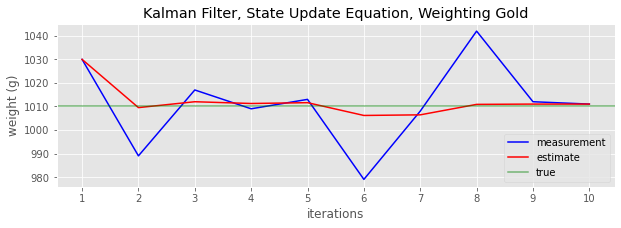We can sample the gold weight from $$\mathcal{N}(1010, 18)$$ 1,000 times and apply the Kalman filter. This sampling from the normal distribution $$\mathcal{N}(1010, 18)$$ simulates the situation as if we were sequentially taking many measurements.

:

measurements = np.random.normal(1010, 18, 1000)
estimates = []
iters = []
x_ij = 1000

for i, z_i in enumerate(measurements):
n = i + 1
alpha_i = 1 / n
x_ii = x_ij + alpha_i * (z_i - x_ij)
x_ij = x_ii

iters.append(n)
estimates.append(x_ii)

measurements = pd.Series(measurements, index=iters)
estimates = pd.Series(estimates, index=iters)


Here, we will plot the measurement, estimate and true values over time. The measurements bounces up and down, but the estimations tend to converge to the true value.

:

fig, ax = plt.subplots(figsize=(10, 3))

_ = measurements.plot(kind='line', ax=ax, color='blue', alpha=0.3, label='measurement')
_ = estimates.plot(kind='line', ax=ax, color='red', label='estimate')
_ = ax.axhline(1010, color='green', alpha=0.5, label='true')
_ = ax.legend()
_ = ax.set_xlabel('iterations')
_ = ax.set_ylabel('weight (g)')
_ = ax.set_title(fr'Kalman Filter, Weighting Gold, $\mu$={measurements.mean():.2f}')

plt.tight_layout()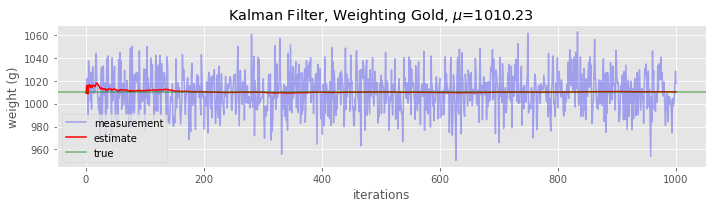Here, we plot the mean absolute error MAE and standard deviation of the MAE. Demote $$w_t$$ as the true weight and $$w_e$$ as the estimated weight, then $$|w_t - w_e|$$ is the absolute error. The MAE is the average over these absolute errors. As we take more and more measurements, the absolute error tends to zero.

:

fig, ax = plt.subplots(figsize=(10, 3))

ae = np.abs(estimates - 1010)
mae = ae.mean()
std = ae.std()

_ = ae.plot(kind='line', ax=ax)
_ = ax.set_xlabel('iterations')
_ = ax.set_ylabel(r'|$w_t$ - $w_e$|')
_ = ax.set_title(r'Absolute difference between true $w_t$ and estimate $w_e$ weight values' +
'\n' +
rf'MAE={mae:.3f}, $\sigma$={std:.3f}')

plt.tight_layout()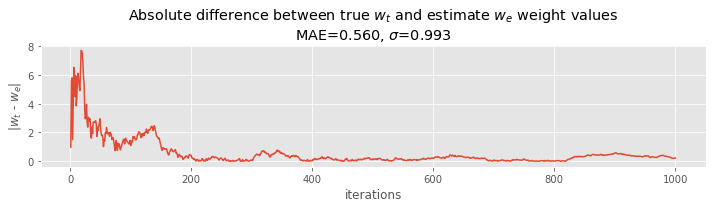## 13.2. Tracking aircraft, constant velocity

In this second example, we are tracking the position of an aircraft with a constant velocity. In the previous example, the gold weight did not change; the dynamic state of the system was constant. Here, the dynamic state of the system changes over time, namely, the position (although the velocity is constant).

How do we estimate the next position?

$$\hat{x}_{n+1,n} = \hat{x}_{n,n} + \Delta{t} v_{n,n}$$

where

• $$\hat{x}_{n,n}$$ is the estimated position in the current time step

• $$\hat{x}_{n+1,n}$$ is the estimated position in the next time step

• $$\Delta{t}$$ is duration (of time, e.g. in seconds; the example sets this to 5)

• $$v_{n,n}$$ is the velocity

Conceptually, where the position of the aircraft will be next depends on where it was plus the time multiplied by the velocity. We also need to estimate the next velocity too. (We diverge from the original article’s notation).

$$\hat{v}_{n+1,n} = \hat{v}_{n,n}$$

Since velocity is constant, the estimation of the next velocity is the same as the previous (the example sets velocity to 40 m/s). The two equations above are rewritten below, and are called the State Extrapolation Equations.

• $$\hat{x}_{n+1,n} = \hat{x}_{n,n} + \Delta{t} v_{n,n}$$

• $$\hat{v}_{n+1,n} = \hat{v}_{n,n}$$

How do we update the position and velocity? The State Update Equation for position is as follows.

$$\hat{x}_{n,n} = \hat{x}_{n,n-1} + \alpha (z_n - \hat{x}_{n,n-1}) = \hat{x}_{n,n-1} + \alpha \Delta{x}$$

The State Update Equation for velocity is as follows.

$$\hat{v}_{n,n} = \hat{v}_{n,n-1} + \beta \frac{z_n - \hat{x}_{n,n-1}}{\Delta{t}} = \hat{v}_{n,n-1} + \beta \frac{\Delta{x}}{\Delta{t}}$$

The weighting parameters $$\alpha$$ and $$\beta$$ do not change in this example. The meaning of $$\alpha$$ and $$\beta$$ is how much we trust the measurements.

• $$\alpha = 1$$ indicates we trust the position measurement

• $$\alpha = 0$$ indicates we do not trust the position measurement

Likewise, for velocity.

• $$\beta = 1$$ indicates we trust the velocity measurement

• $$\beta = 0$$ indicates we do not trust the velocity measurement

The two equations below are called $$\alpha$$ - $$\beta$$ track update equations or $$\alpha$$ - $$\beta$$ track filtering equations.

• $$\hat{x}_{n,n} = \hat{x}_{n,n-1} + \alpha (z_n - \hat{x}_{n,n-1})$$

• $$\hat{v}_{n,n} = \hat{v}_{n,n-1} + \beta \frac{z_n - \hat{v}_{n,n-1}}{\Delta{t}}$$

These two equations are also called the g-h filter where $$g$$ is substituting for $$\alpha$$ and $$h$$ for $$\beta$$.

:

mea = [30110, 30265, 30740, 30750, 31135, 31015, 31180, 31610, 31960, 31865]
data = []
itr = []

a = 0.2
b = 0.1
t = 5

# initialization
p_ii = 30000
v_ii = 40

# State Extrapolation Equations
p_ji = p_ii + t * v_ii
v_ji = v_ii

itr.append(0)
data.append({'pos_e': p_ii, 'vel_e': v_ii, 'pos_p': p_ji, 'vel_p': v_ji, 'pos_m': p_ii})

for i, z_i in enumerate(mea):
# State Update Equations
p_ii = p_ji + a * (z_i - p_ji)
v_ii = v_ji + b * (z_i - p_ji) / t

# State Extrapolation Equations
p_ji = p_ii + t * v_ii
v_ji = v_ii

time = t * (i + 1)
itr.append(time)

data.append({'pos_e': p_ii, 'vel_e': v_ii, 'pos_p': p_ji, 'vel_p': v_ji, 'pos_m': z_i})

data = pd.DataFrame(data, index=itr)
data

:

pos_e vel_e pos_p vel_p pos_m
0 30000.000000 40.000000 30200.000000 40.000000 30000
5 30182.000000 38.200000 30373.000000 38.200000 30110
10 30351.400000 36.040000 30531.600000 36.040000 30265
15 30573.280000 40.208000 30774.320000 40.208000 30740
20 30769.456000 39.721600 30968.064000 39.721600 30750
25 31001.451200 43.060320 31216.752800 43.060320 31135
30 31176.402240 39.025264 31371.528560 39.025264 31015
35 31333.222848 35.194693 31509.196312 35.194693 31180
40 31529.357050 37.210767 31715.410882 37.210767 31610
45 31764.328706 42.102549 31974.841450 42.102549 31960
50 31952.873160 39.905720 32152.401760 39.905720 31865

Below, we plot the estimated $$x_e$$, measured $$x_m$$ and true $$x_t$$ positions over time.

:

fig, ax = plt.subplots(figsize=(10, 5))

_ = data.pos_e.plot(kind='line', color='red', label=r'$x_e$', ax=ax)
_ = data.pos_m.plot(kind='line', color='blue', label=r'$x_m$', ax=ax)
_ = ax.plot([0, 1], [0, 1], color='green', label=r'$x_t$', alpha=0.5, transform=ax.transAxes)
_ = ax.set_xticks(data.index)
_ = ax.set_xticklabels(data.index)
_ = ax.legend()
_ = ax.set_xlabel('time (s)')
_ = ax.set_ylabel(r'$x$, position')
_ = ax.set_title('Position Tracking')

plt.tight_layout()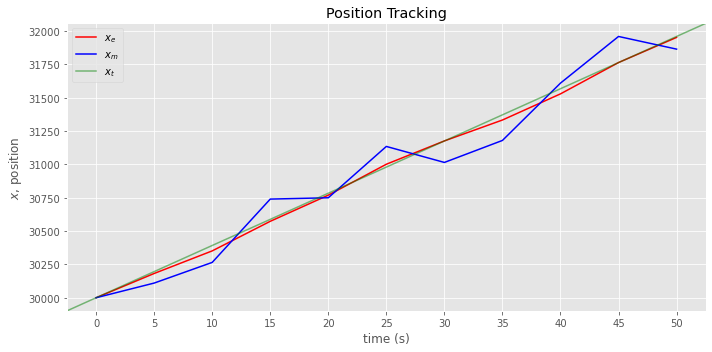Here, we plot the estimated $$v_e$$ and true $$v_t$$ velocities over time.

:

fig, ax = plt.subplots(figsize=(10, 5))

_ = data.vel_e.plot(kind='line', color='red', label=r'$v_e$', ax=ax)
_ = ax.axhline(40, color='green', alpha=0.5, label=r'$v_t$')
_ = ax.set_xticks(data.index)
_ = ax.set_xticklabels(data.index)
_ = ax.legend()
_ = ax.set_xlabel('time (s)')
_ = ax.set_ylabel(r'$v$, velocity')
_ = ax.set_title('Velocity Estimation')

plt.tight_layout()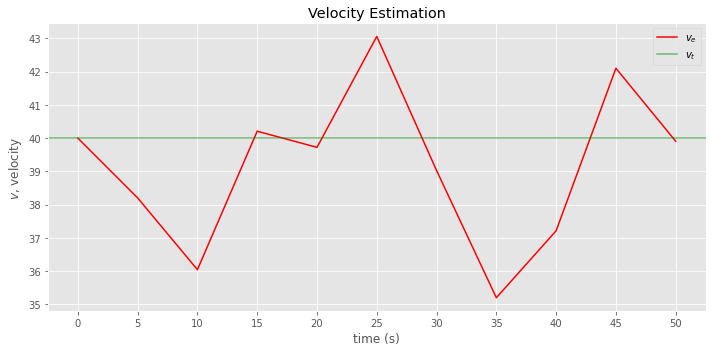Let’s simulate position data according to the following.

$$x_{n+1,n} = x_{n,n} + \Delta{t} \mathcal{N}(40, 2.4)$$

:

dt = 5
t = np.arange(0, 200 + dt, dt)
N = len(t)

pos = 
vel = 

for n in range(N):
p = pos[n] + dt * vel[n]
v = np.random.normal(40, 2.4)
pos.append(p)
vel.append(v)


Now, let’s apply the State Extrapolation and Updating Equations to estimate the positions.

:

mea = pos
data = []
itr = []

a = 0.2
b = 0.1
t = 5

# initialization
p_ii = 30000
v_ii = 40

# State Extrapolation Equations
p_ji = p_ii + t * v_ii
v_ji = v_ii

itr.append(0)
data.append({'pos_e': p_ii, 'vel_e': v_ii, 'pos_p': p_ji, 'vel_p': v_ji, 'pos_m': p_ii})

for i, z_i in enumerate(mea):
# State Update Equations
p_ii = p_ji + a * (z_i - p_ji)
v_ii = v_ji + b * (z_i - p_ji) / t

# State Extrapolation Equations
p_ji = p_ii + t * v_ii
v_ji = v_ii

time = t * (i + 1)
itr.append(time)

data.append({'pos_e': p_ii, 'vel_e': v_ii, 'pos_p': p_ji, 'vel_p': v_ji, 'pos_m': z_i})

data = pd.DataFrame(data, index=itr)

:

fig, ax = plt.subplots(figsize=(10, 5))

_ = data[data.index < 200].pos_e.plot(kind='line', color='red', label=r'$x_e$', ax=ax)
_ = data[data.index < 200].pos_m.plot(kind='line', color='blue', label=r'$x_m$', alpha=0.5, ax=ax)
_ = ax.plot([0, 1], [0, 1], color='green', label=r'$x_t$', alpha=0.5, transform=ax.transAxes)
_ = ax.legend()
_ = ax.set_xlabel('time (s)')
_ = ax.set_ylabel(r'$x$, position')
_ = ax.set_title('Position Tracking')

plt.tight_layout()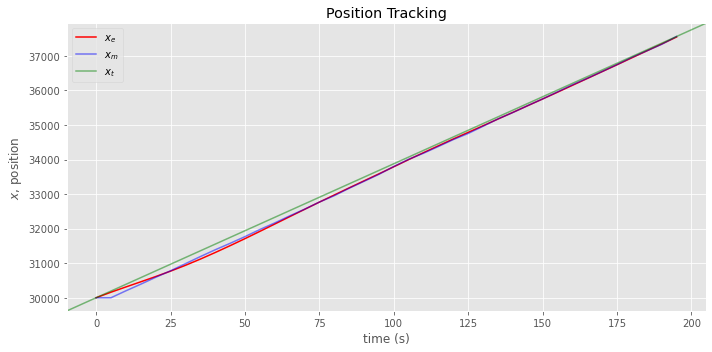:

fig, ax = plt.subplots(figsize=(10, 5))

_ = data.vel_e.plot(kind='line', color='red', label=r'$v_e$', ax=ax)
_ = ax.axhline(40, color='green', alpha=0.5, label=r'$v_t$')
_ = ax.legend()
_ = ax.set_xlabel('time (s)')
_ = ax.set_ylabel(r'$v$, velocity')
_ = ax.set_title('Velocity Estimation')

plt.tight_layout()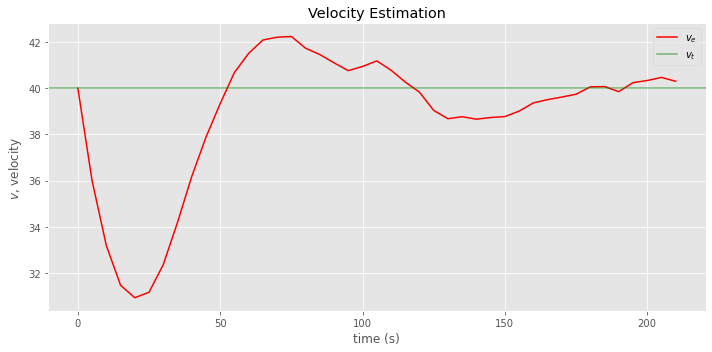## 13.3. Tracking aircraft, changing velocity and acceleration

In the third example, the position of an aircraft with changing velocity and acceleration is tracked using the model in second example. This example serves to illustrate that if a model does not correctly capture the dynamics, then lag error results. The lag error goes by many names.

• Dynamic error

• Systematic error

• Bias error

• Truncation error

These are the true values of acceleration, velocity and position.

:

tim = [0, 5, 10, 15, 20, 25, 30, 35, 40, 45, 50]
acc = [0, 0, 0, 0, 8, 8, 8, 8, 8, 8, 8]
vel = [50, 50, 50, 50, 90, 130, 170, 210, 250, 290, 330]
pos = [30000, 30250, 30500, 30750, 31200, 31850, 32700, 33750, 35000, 36450, 38100]

acc = pd.Series(acc, index=tim)
vel = pd.Series(vel, index=tim)
pos = pd.Series(pos, index=tim)


Now we take measurements and make estimations.

:

mea = [30160, 30365, 30890, 31050, 31785, 32215, 33130, 34510, 36010, 37265]
data = []
itr = []

a = 0.2
b = 0.1
t = 5

# initialization
p_ii = 30000
v_ii = 50

# State Extrapolation Equations
p_ji = p_ii + t * v_ii
v_ji = v_ii

itr.append(0)
data.append({'pos_e': p_ii, 'vel_e': v_ii, 'pos_p': p_ji, 'vel_p': v_ji, 'pos_m': p_ii})

for i, z_i in enumerate(mea):
# State Update Equations
p_ii = p_ji + a * (z_i - p_ji)
v_ii = v_ji + b * (z_i - p_ji) / t

# State Extrapolation Equations
p_ji = p_ii + t * v_ii
v_ji = v_ii

time = t * (i + 1)
itr.append(time)

data.append({'pos_e': p_ii, 'vel_e': v_ii, 'pos_p': p_ji, 'vel_p': v_ji, 'pos_m': z_i})

data = pd.DataFrame(data, index=itr)
data

:

pos_e vel_e pos_p vel_p pos_m
0 30000.000000 50.000000 30250.000000 50.000000 30000
5 30232.000000 48.200000 30473.000000 48.200000 30160
10 30451.400000 46.040000 30681.600000 46.040000 30365
15 30723.280000 50.208000 30974.320000 50.208000 30890
20 30989.456000 51.721600 31248.064000 51.721600 31050
25 31355.451200 62.460320 31667.752800 62.460320 31785
30 31777.202240 73.405264 32144.228560 73.405264 32215
35 32341.382848 93.120693 32806.986312 93.120693 33130
40 33147.589050 127.180967 33783.493882 127.180967 34510
45 34228.795106 171.711089 35087.350550 171.711089 36010
50 35522.880440 215.264078 36599.200830 215.264078 37265
:

fig, ax = plt.subplots(figsize=(10, 5))

_ = data.pos_e.plot(kind='line', color='red', label=r'$x_e$', ax=ax)
_ = data.pos_m.plot(kind='line', color='blue', label=r'$x_m$', ax=ax)
_ = pos.plot(kind='line', color='green', label=r'$x_t$', ax=ax)
_ = ax.set_xticks(data.index)
_ = ax.set_xticklabels(data.index)
_ = ax.legend()
_ = ax.set_xlabel('time (s)')
_ = ax.set_ylabel(r'$x$, position')
_ = ax.set_title('Position Tracking')

plt.tight_layout()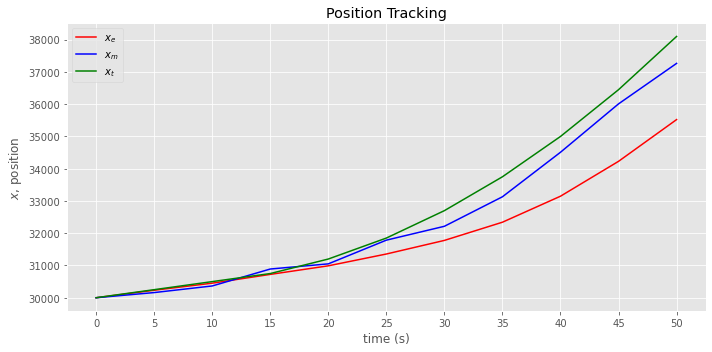:

fig, ax = plt.subplots(figsize=(10, 5))

_ = data.vel_e.plot(kind='line', color='red', label=r'$v_e$', ax=ax)
_ = vel.plot(kind='line', color='green', label=r'$v_t$', ax=ax)
_ = ax.set_xticks(data.index)
_ = ax.set_xticklabels(data.index)
_ = ax.legend()
_ = ax.set_xlabel('time (s)')
_ = ax.set_ylabel(r'$v$, velocity')
_ = ax.set_title('Velocity Estimation')

plt.tight_layout()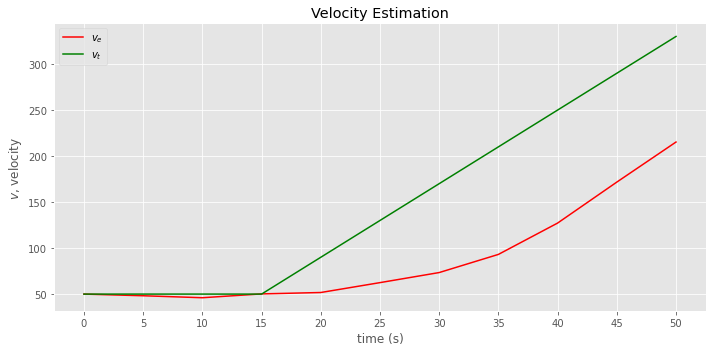## 13.4. Tracking aircraft, $$\alpha$$ - $$\beta$$ - $$\gamma$$ filter

To properly track the aircraft, our model must consider the changing acceleration. We should use the $$\alpha$$ - $$\beta$$ - $$\gamma$$ filter (or, g-h-k filter). The State Extrapolation Equations are given as follows.

• $$\hat{x}_{n+1,n} = \hat{x}_{n,n} + \hat{v}_{n,n} \Delta{t} + \hat{a}_{n,n} \frac{\Delta t^2}{2}$$

• $$\hat{v}_{n+1,n} = \hat{v}_{n,n} + \hat{a} \Delta t$$

• $$\hat{a}_{n+1,n} = \hat{a}_{n,n}$$

The State Update Equations are given as follows.

• $$\hat{x}_{n,n} = \hat{x}_{n,n-1} + \alpha \Delta x$$

• $$\hat{v}_{n,n} = \hat{v}_{n,n-1} + \beta \frac{ \Delta x}{ \Delta t}$$

• $$\hat{a}_{n,n} = \hat{a}_{n,n-1} + \gamma \frac{ \Delta x}{0.5 \Delta t^2}$$

:

mea = [30160, 30365, 30890, 31050, 31785, 32215, 33130, 34510, 36010, 37265]
data = []
itr = []

a = 0.5
b = 0.4
c = 0.1
t = 5

# initialization
p_ii = 30000
v_ii = 50
a_ii = 0

# State Extrapolation Equations
p_ji = p_ii + (t * v_ii) + (0.5 * a_ii * t**2)
v_ji = v_ii + (a_ii * t)
a_ji = a_ii

itr.append(0)
data.append({
'pos_e': p_ii,
'vel_e': v_ii,
'acc_e': a_ii,
'pos_p': p_ji,
'vel_p': v_ji,
'acc_p': a_ji,
'pos_m': p_ii
})

for i, z_i in enumerate(mea):
# State Update Equations
dx = z_i - p_ji
p_ii = p_ji + a * dx
v_ii = v_ji + b * (dx / t)
a_ii = a_ji + c * (dx / 0.5 / t**2)

# State Extrapolation Equations
p_ji = p_ii + (t * v_ii) + (0.5 * a_ii * t**2)
v_ji = v_ii + (a_ii * t)
a_ji = a_ii

time = t * (i + 1)
itr.append(time)

data.append({
'pos_e': p_ii,
'vel_e': v_ii,
'acc_e': a_ii,
'pos_p': p_ji,
'vel_p': v_ji,
'acc_p': a_ji,
'pos_m': z_i
})

data = pd.DataFrame(data, index=itr)
data

:

pos_e vel_e acc_e pos_p vel_p acc_p pos_m
0 30000.00000 50.00000 0.000000 30250.0000 50.00000 0.000000 30000
5 30205.00000 42.80000 -0.720000 30410.0000 39.20000 -0.720000 30160
10 30387.50000 35.60000 -1.080000 30552.0000 30.20000 -1.080000 30365
15 30721.00000 57.24000 1.624000 31027.5000 65.36000 1.624000 30890
20 31038.75000 67.16000 1.804000 31397.1000 76.18000 1.804000 31050
25 31591.05000 107.21200 4.907200 32188.4500 131.74800 4.907200 31785
30 32201.72500 133.87200 5.119600 32935.0800 159.47000 5.119600 32215
35 33032.54000 175.06360 6.678960 33991.3450 208.45840 6.678960 33130
40 34250.67250 249.95080 10.828200 35635.7790 304.09180 10.828200 34510
45 35822.88950 334.02948 13.821968 37665.8115 403.13932 13.821968 36010
50 37465.40575 371.07440 10.615476 39453.4712 424.15178 10.615476 37265
:

fig, ax = plt.subplots(figsize=(10, 5))

_ = data.pos_e.plot(kind='line', color='red', label=r'$x_e$', ax=ax)
_ = data.pos_m.plot(kind='line', color='blue', label=r'$x_m$', ax=ax)
_ = pos.plot(kind='line', color='green', label=r'$x_t$', ax=ax)
_ = ax.set_xticks(data.index)
_ = ax.set_xticklabels(data.index)
_ = ax.legend()
_ = ax.set_xlabel('time (s)')
_ = ax.set_ylabel(r'$x$, position')
_ = ax.set_title('Position Tracking')

plt.tight_layout()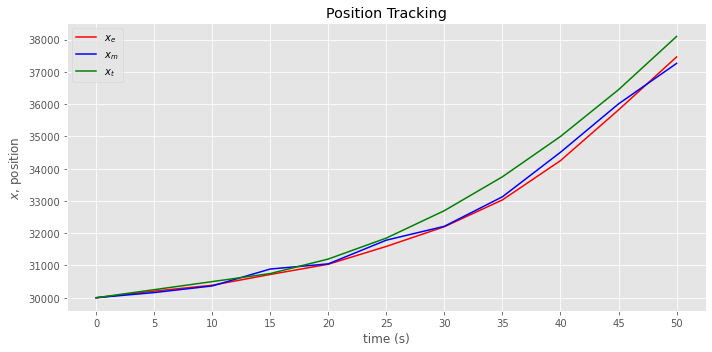:

fig, ax = plt.subplots(figsize=(10, 5))

_ = data.vel_e.plot(kind='line', color='red', label=r'$v_e$', ax=ax)
_ = vel.plot(kind='line', color='green', label=r'$v_t$', ax=ax)
_ = ax.set_xticks(data.index)
_ = ax.set_xticklabels(data.index)
_ = ax.legend()
_ = ax.set_xlabel('time (s)')
_ = ax.set_ylabel(r'$v$, velocity')
_ = ax.set_title('Velocity Estimation')

plt.tight_layout()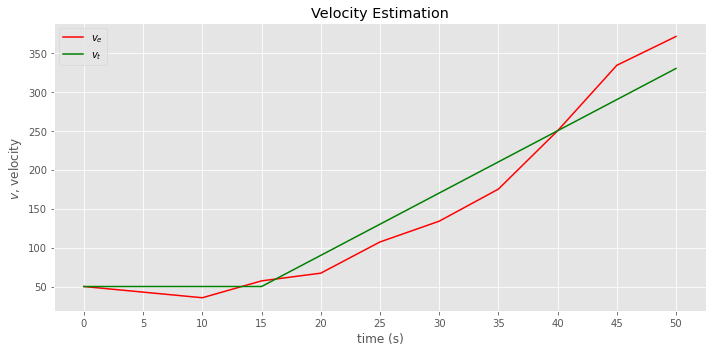:

fig, ax = plt.subplots(figsize=(10, 5))

_ = data.acc_e.plot(kind='line', color='red', label=r'$a_e$', ax=ax)
_ = acc.plot(kind='line', color='green', label=r'$a_t$', ax=ax)
_ = ax.set_xticks(data.index)
_ = ax.set_xticklabels(data.index)
_ = ax.legend()
_ = ax.set_xlabel('time (s)')
_ = ax.set_ylabel(r'$a$, acceleration')
_ = ax.set_title('Acceleration Estimation')

plt.tight_layout()